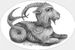# QlikView App Dev

Discussion Board for collaboration related to QlikView App Development.

Announcements
Join us at the Cloud Data and Analytics Tour! REGISTER TODAY
cancel
Showing results for
Search instead for
Did you mean:Contributor II

## Add new column and fill conditionally

I have the following code to load all of my files into qlikview:

Data:

// Dummy load so we can use concatenate below

LOAD 0 as dummy AutoGenerate 0;

Concatenate (Data)

LOAD
Col1,
Col2,
Col3,
Col4

FROM

[foo\*.xlsx]

(ooxml, embedded labels)
;DROP FIELD dummy; // Drop dummy field

Now i want to add a new column at the end but it is conditionally on a previous column.

Pseudocode would look like this:

Define_Array \$CONDITION1 = 'String1','String2','String3'

Define_Array \$CONDITION2 = 'String4','String5','String6'

Add Col5 to data //Add an empty column

If Col3 = \$CONDITION1 then //So if Col3 is any of the string from the array
Col5 = 'Cond1'

ElseIf Col3 = \$CONDITION2 then //So if Col3 is any of the string from the array
Col5 = 'Cond2'

Else
Col5 = 'N/A'

EndIf

So that in the end i have all my data from my excel files but added one new column at the end

Labels (3)

• ### table

1 Solution

Accepted SolutionsMaster

One solution is:

``````Set vCONDITION1 = 'String1','String2','String3';

Set vCONDITION2 = "'String4','String5','String6'";

tab1:
LOAD *, If(Match(Col1,\$(vCONDITION1)),'Cond1',
If(Match(Col1,\$(vCONDITION2)),'Cond2',
'N/A'
)
) As Col5
;
Load 'String'&Round(Rand()*10) As Col1,
Pick(Ceil(3*Rand()),'X','Y','Z') as Col2,
Round(1000*Rand()*Rand()) as Col3,
Round(  10*Rand()*Rand()) as Col4
AutoGenerate 10;``````1 ReplyMaster

One solution is:

``````Set vCONDITION1 = 'String1','String2','String3';

Set vCONDITION2 = "'String4','String5','String6'";

tab1:
LOAD *, If(Match(Col1,\$(vCONDITION1)),'Cond1',
If(Match(Col1,\$(vCONDITION2)),'Cond2',
'N/A'
)
) As Col5
;
Load 'String'&Round(Rand()*10) As Col1,
Pick(Ceil(3*Rand()),'X','Y','Z') as Col2,
Round(1000*Rand()*Rand()) as Col3,
Round(  10*Rand()*Rand()) as Col4
AutoGenerate 10;``````